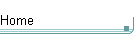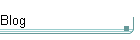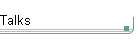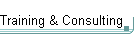GotW #52On theblogNovember 4: Other Concurrency Sessions at PDC November 3 October 26: Hoare on Testing October 23This is the original GotW problem and solution substantially as posted to Usenet. See the book More Exceptional C++ (Addison-Wesley, 2002) for the most current solution to this GotW issue. The solutions in the book have been revised and expanded since their initial appearance in GotW. The book versions also incorporate corrections, new material, and conformance to the final ANSI/ISO C++ standard.

## Extending the Standard Library - Part II Difficulty: 7 / 10

Following up from the introduction given in #51, we now examine "stateful" predicates. What are they? When are they useful? How compatible are they with standard containers and algorithms?### Problem

#### JG Question

1. What are predicates, and how are they used in STL? Give an example.

#### Guru Questions

2. When would a "stateful" predicate be useful? Give examples.

3. What requirements on algorithms are necessary in order to make stateful predicates work correctly?### Solution

1. What are predicates, and how are they used in STL?

A predicate is a pointer to a function, or a functor (an object that supplies the function call operator, operator()()), that an algorithm can apply to each element it operates on. Algorithms normally use the predicate to make a decision about the element, so a predicate 'pred' should work correctly when used as follows:

```    //  Example 1(a).
//
if( pred( *first ) )
{
/* ... */
}```

As you can see from this example, 'pred' should return a value that can be tested as true. Note that a predicate is not allowed to use any non-const function through the dereferenced iterator.

Some predicates are binary; that is, they take two dereferenced iterators as arguments. This means that a binary predicate 'bpred' should work correctly when used as follows:

```    //  Example 1(b).
//
if( bpred( *first1, *first2 ) )
{
/* ... */
}```

Give an example.

Consider the following implementation of the standard algorithm find_if():

```    //  Example 1(c).
//
template<class Iter, class Pred> inline
Iter find_if( Iter first, Iter last, Pred pred )
{
for( ; first != last; ++first )
{
if( pred(*first) )
{
break;
}
}
return (first);
}```

This implementation of the algorithm visits every element in the range [first, last) in order, applying the predicate function pointer or object 'pred' to each element. If there is an element for which the predicate evaluates to true, find_if() returns an iterator pointing to the first such element. Otherwise, find_if() returns 'last' to signal that an element satisfying the predicate was not found.

We can use find_if() with a function pointer predicate as follows:

```    //  Example 1(d): Using find_if() with a
//                function pointer.
//
bool GreaterThanFive( int i )
{
return i > 5;
}```
```    bool IsAnyElementGreaterThanFive( vector<int>& v )
{
return find_if( v.begin(), v.end(), GreaterThanFive )
!= v.end();
}```

Here's the same example, only using find_if() with a functor instead of a free function:

```    //  Example 1(e): Using find_if() with a
//                functor.
//
struct GreaterThanFive
{
bool operator()( int i )
{
return i > 5;
}
};```
```    bool IsAnyElementGreaterThanFive( vector<int>& v )
{
return find_if( v.begin(), v.end(), GreaterThanFive() )
!= v.end();
}```

In this example, there's not much benefit to using a functor over a free function, is there? But this leads us nicely into our guru questions, where the functor starts showing much greater flexibility:

2. When would a "stateful" predicate be useful? Give examples.

Continuing on from examples 1(d) and 1(e), here's something that a free function can't do as easily without using something like a static variable:

```    //  Example 2(a): Using find_if() with a
//                more general functor.
//
class GreaterThan
{
public:
GreaterThan( int value ) : value_( value ) { }
bool operator()( int i ) const
{
return i > value_;
}```
```    private:
const int value_;
};```
```    bool IsAnyElementGreaterThanFive( vector<int>& v )
{
return find_if( v.begin(), v.end(), GreaterThan(5) )
!= v.end();
}```

This GreaterThan predicate has member data that remembers a value, in this case the value that it should compare each element against. You can already see that this version is much more usable -- and, therefore, reusable -- than the special-purpose code in examples 1(d) and 1(e), and a lot of the power comes from the ability to store local information inside the object like this. (This object is not "stateful" yet, though, because once constructed it does not change.)

Taking it one step further, we end up with something even more generalized:

```    //  Example 2(b): Using find_if() with a
//                fully general functor.
//
template<class T>
class GreaterThan
{
public:
GreaterThan( T value ) : value_( value ) { }
bool operator()( const T& t ) const
{
return t > value_;
}

private:
const T value_;
};

bool IsAnyElementGreaterThanFive( vector<int>& v )
{
return find_if( v.begin(), v.end(), GreaterThan<int>(5) )
!= v.end();
}```

So we can see some usability benefits from using predicates that store value.

#### The Next Step: Stateful Predicates

The predicates in both examples 2(a) and 2(b) have an important property: Copies are equivalent. That is, if you make a copy of a GreaterThan<int> object, it behaves in all respects just like the original one and can be used interchangeably. This turns out to be important, as we shall see in Question #3.

Some people have tried to write "stateful" predicates that go further by changing as they're used; that is, the result of applying a predicate depends on its history of previous applications.

Examples of such stateful predicates appear in books. In particular, people have tried to write predicates that keep track of various information about the elements that they were applied to. For example, people have proposed predicates that remember the values of the objects they were applied to in order to perform calculations (e.g., a predicate that returns true as long as the average of the values it was applied to so far is more than 50, or the total is less than 100, and so on). We just saw a specific example of this kind of stateful predicate in GotW #51, Question #3:

```    //  Example 2(c)
//  (From GotW #51, Question #3)
//
//  Method 2: Write a functor which returns true
//            the nth time it's applied, and use
//            that as a predicate for remove_if.
//
template<class T>
class FlagNth
{
public:
FlagNth( size_t n ) : i_(0), n_(n) { }
bool operator()( const T& ) { return i_++ == n_; }
private:
size_t       i_;
const size_t n_;
};```

Stateful predicates are sensitive to the way they are applied to elements in the range being operated on: This one in particular depends on both the number of times it has been applied (this should be obvious), and on the order in which it is applied to the elements in the range (if used in conjunction with something like remove_if(); this may be less obvious at first).

The major difference between predicates that are stateful and those that aren't are that, for stateful predicates, copies are NOT equivalent. Clearly an algorithm couldn't make a copy of a FlagNth<T> object, and apply one object to some elements and the other object to other elements. That wouldn't give the expected results at all, because the two predicate objects would update their counts independently and neither would be able to flag the correct n-th element; each could only flag the n-th element that it happened to be applied to.

The problem is that, in GotW #51's Question #3, our Method 2 tried to use a FlagNth<T> object in just such a way (possibly):

```    // Example invocation
... remove_if( v.begin(), v.end(), FlagNth<int>(3) ) ...```

"Looks reasonable, and I've used this technique," you say? "I just read a C++ book that demonstrates this technique, so it must be fine," you say? Well, the truth is that this technique may happen to work on your implementation (or on the implementation that the author of book with the error in it was using), but it is NOT guaranteed to work portably on all implementations, or even on the next version of the implementation you are (or that author is) using now.

Let's see why, by examining remove_if() in a little more detail in Question #3:

3. What requirements on algorithms are necessary in order to make stateful predicates work correctly?

For stateful predicates to be really useful with an algorithm, the algorithm must generally guarantee two things about how it uses the predicate:

a) that it does not make copies of the predicate (that is, it should consistently use the same object that it was given); and

b) that the predicate is applied to the elements in the range in some known order (usually, first to last).

Alas, the standard does not require that the standard algorithms meet these two guarantees (yes, even though stateful predicates have appeared in books; in a battle between the standard and a book, the standard wins). The standard does mandate other things for standard algorithms, such as the performance complexity and the number of times a predicate is applied, but in particular it never specifies requirement (a) for any algorithm.

For example, consider std::remove_if():

a) It's common for standard library implementations implement remove_if() in terms of find_if(), and pass the predicate along to find_if() by value. This will make the predicate behave unexpectedly, because the predicate object actually passed to remove_if() is not necessarily applied once to every element in the range... "the predicate object _or a copy of the predicate object_" is what is guaranteed to be applied once to every element. This is because a conforming remove_if() is allowed to assume that copies of the predicate are equivalent. [As an aside, note that this means that auto_ptrs can never be used as predicates.]

b) The standard requires that the predicate supplied to remove_if() be applied exactly "last - first" times, but that doesn't mean that the predicate will necessarily be applied to the elements in any given order. It's possible (if a little obnoxious) to write a conforming implementation of remove_if() that doesn't apply the predicate to the elements in order. The point is that, if it's not required by the standard, you can't portably depend on it.

"Well," you ask, "isn't there ANY way to make stateful predicates like FlagNth<> work reliably with the standard algorithms?" Unfortunately, the answer is No.

...All right, all right, quiet down! I can already hear the howls from the folks who write predicates that use reference-counting techniques to solve the predicate-copying problem (a) above. Yes, you can share the predicate state so that a predicate can be safely copied without changing its semantics when it is applied to objects. The following code uses this technique (for a suitable CountedPtr template; follow-up question: provide a suitable implementation of CountedPtr):

```    //  Example 3(a): A (partial) solution that
//                shares state between copies.
//
template<class T>
struct FlagNthImpl
{
FlagNthImpl( size_t nn ) : i(0), n(nn) { }
size_t       i;
const size_t n;
};

template<class T>
class FlagNth
{
public:
FlagNth( size_t n )
: pimpl_( new FlagNthImpl<T>( n ) )
{
}

bool operator()( const T& )
{
return (pimpl_->i)++ == pimpl_->n;
}
private:
CountedPtr<FlagNthImpl<T> > pimpl_;
};```

But this doesn't (and can't) solve the ordering problem (b) above. That is, you the programmer are entirely dependent on the order in which the predicate is applied by the algorithm. There's no way around this, not even with fancy pointer techniques, unless the algorithm itself guarantees a traversal order.

#### Follow-Up Question

The follow-up question, above, was to provide a suitable implementation of CountedPtr, a smart pointer for which making a new copy just points at the same representation, and the last copy to be destroyed cleans up the allocated object. Here is a skeletal one I just made up on the spot:

```    template<class T>
class CountedPtr
{
private:
struct Impl
{
Impl( T* pp ) : p( pp ), refs( 1 ) { }

~Impl() { delete p; }

T*     p;
size_t refs;
};
Impl* impl_;

public:
CountedPtr( T* p )
: impl_( new Impl( p ) )
{
}

~CountedPtr()
{
Decrement();
}

CountedPtr( const CountedPtr& other )
: impl_( other.impl_ )
{
Increment();
}

CountedPtr& operator=( const CountedPtr& other )
{
if( impl_ != other.impl_ )
{
Decrement();
impl_ = other.impl_;
Increment();
}
return *this;
}

T* operator->()
{
return impl_->p;
}

private:
void Decrement()
{
if( --(impl_->refs) == 0 )
{
delete impl_;
}
}

void Increment()
{
++(impl_->refs);
}
};```

#### Notes

1. As Astute Reader John D. Hickin so elegantly describes it: "The input [first, last) is somewhat like the tape fed to a Turing machine and the stateful predicate is like a program."